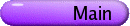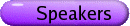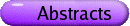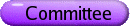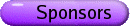Differentiable Dynamics and Smooth Ergodic Theory
Org: Giovanni Forni and Konstantin Khanin (Toronto)
[PDF]

JAYADEV ATHREYA, Yale University, Dept. of Mathematics, 10 Hillhouse Ave, New Haven, CT 06511
A lattice point problem in Teichmuller space
[PDF]

Let Tg denote the Teichmuller space of marked compact Riemann surfaces of genus g. Let Gg be the associated mapping class group. Let Qg be the unit co-tangent bundle to Tg, that is, the space of (marked) unit area holomorphic quadratic differentials. Let p: Qg ® Tg be the natural projection, and let m denote the natural Gg-invariant measure on Qg. Then m = p* m is a measure on Teichmuller space. In joint work with Bufetov, Eskin, and Mirzakhani, we calculate the asymptotics of

• |Gg x ÈB(y,R)|, for x,y Î Tg, where B(y,R) denotes the ball of radius R in the Teichmuller metric;

• m ( B(x,R) ).

PAVEL BATCHOURINE, University of Toronto
On ergodicity of multidimensional dispersing billiards
[PDF]

Ergodic theory of dispersing billiards was developed in 1970s-1980s. An important part of the theory is the analysis of the structure of the sets where the billiard map is discontinuous. They were assumed to be smooth manifolds till recently, when a new pathological type of behaviour of these sets was found. Thus a reconsideration of earlier arguments was needed. I'll show that at least in a generic situation the earlier proofs of ergodicity of dispersing billiards can be recovered.

BASSAM FAYAD, Paris 13, av. JB Clément, Villetaneuse, France
Smooth linearization of commuting circle diffeomorphisms
[PDF]

We show that smooth commuting circle diffeomorphisms with simultaneously diophantine rotation numbers are smoothly conjugated to rotations.

VADIM KALOSHIN, Department of Mathematics, The Pennsylvania State University, University Park, PA 16802, USA
Hausdorff dimension of oscillatory motions for the 3-body problem
[PDF]

Consider the classical 3-body problem mutually attracted by Newton gravitation. Call motions oscillatory if at time tends to infinity limsup of maximal distance among the bodies is infinite, while liminf is finite. In the '50s Sitnikov presented the first rigorous example of oscillatory motions for the so-called restricted 3-body problem. Later in the '60s Alexeev extended this example to the 3-body problem. A long-standing conjecture, probably going back to Kolmogorov, is that oscillatory motions have measure zero. We show that for the Sitnikov example and for the so-called restricted planar circular 3-body problem these motions often have full Hausdorff dimension.

This is a joint work with Anton Gorodetski.

FEDERICO RODRIGUEZ-HERTZ, IMERL, Uruguay
Partial hyperbolicity and ergodicity in dimension three
[PDF]

In [HHU] we proved the Pugh-Shub conjecture for conservative partially hyperbolic diffeomorphisms with one-dimensional center. That is, stably ergodic diffeomorphisms are dense among the conservative partially hyperbolic ones. Can we describe this abundance of ergodicity more accurately?

More precisely:

Problem    Which 3-dimensional manifolds support a non-ergodic partially hyperbolic diffeomorphism?

We conjecture that the answer to this question is that the only such manifolds are the mapping tori of diffeomorphisms commuting with an Anosov one. In the other cases, being partially hyperbolic would automatically imply ergodicity. We prove this for a family of manifolds:

Theorem    Let f : N® N be a conservative partially hyperbolic C2 diffeomorphism where N ¹ T3 is a compact 3-dimensional nilmanifold. Then, f is ergodic.

Sacksteder [Sa] proved that certain affine diffeomorphisms of nilmanifolds are ergodic. These examples are partially hyperbolic.

Some of our results apply to other manifolds and we obtain, for instance, that every conservative partially hyperbolic diffeomorphism of S3 is ergodic but this is probably a theorem about the empty set.

This is a joint work with María Alejandra Rodriguez Hertz and Raúl Ures.

## References

[BW]
K. Burnsa and A. Wilkinson, On the ergodicity of partially hyperbolic systems. Ann. Math., to appear.

[PS]
C. Pugh and M. Shub, Stable ergodicity and julienne quasiconformality. J. Eur. Math. Soc. 2(2000), 1-52.

[HHU]
F. Rodriguez Hertz, M. Rodriguez Hertz and R. Ures, Accessibility and stable ergodicity for partially hyperbolic diffeomorphisms with 1d-center bundle. Preprint.

[Sa]
R. Sacksteder, Strongly mixing transformations. In: 1970 Global Analysis, Proc. Sympos. Pure Math. XIV, Berkeley, Calif., 1968, 245-252.

MIKE SHUB, University of Toronto
Non-Zero Lyapunov Exponents in Families of Dynamical Systems
[PDF]

We compare the average Lyapunov exponent in families of twist maps and one dimensional expanding maps to the Lyapunov exponents of random products chosen i.i.d. from these families. For some families we prove that the average exponent is bigger than the random exponent. In other cases the provable and experimental results are very suggestive.

Different portions of the work surveyed are joint work with Francois Ledrappier, Rafael de la LLave, Enrique Pujals, Leonel Robert, Carles Simo and Amie Wilkinson.

CORINNA ULCIGRAI, Princeton University, Mathematics Department, Fine Hall, Washington Rd, Princeton, NJ 08544
Mixing of flows over interval exchange maps
[PDF]

We consider suspension flows over interval exchange transformations, under a roof function with logarithmic singularities. As a motivation, such flows arise as minimal components of flows on surfaces given by multi-valued Hamiltonians. We prove that if the roof function has an asymmetric logarithmic singularity, the suspension flow is strongly mixing for a full measure set of interval exchanges. This generalizes a result by Khanin and Sinai for flows over rotations of the circle. In the proof we use a recent result by Avila-Gouzel-Yoccoz.

AMIE WILKINSON, Northwestern University, Evanston, IL, USA
Asymmetrical diffeomorphisms
[PDF]

Which diffeomorphisms of a compact manifold M commute with no other diffeomorphisms (except their own powers)? Smale asked if such highly asymmetrical diffeomorphisms are typical, in that they are dense in the Cr topology on the space of Cr diffeomorphisms Diffr(M). In this talk I will explain the recent (positive) solution to Smale's question for C1 symplectomorphisms and volume-preserving diffeomorphisms. I will also discuss progress on the general case.

This is joint work with Christian Bonatti and Sylvain Crovisier.